Example 40.17 Linear Inference Based on Summary Data

The GLIMMIX procedure has facilities for multiplicity-adjusted inference through the ADJUST= and STEPDOWN options in the ESTIMATE, LSMEANS, and LSMESTIMATE statements. You can employ these facilities to test linear hypotheses among parameters even in situations where the quantities were obtained outside the GLIMMIX procedure. This example demonstrates the process. The basic idea is to prepare a data set containing the estimates of interest and a data set containing their covariance matrix. These are then passed to the GLIMMIX procedure, preventing updating of the parameters, essentially moving directly into the post-processing stage as if estimates with this covariance matrix had been produced by the GLIMMIX procedure.

The final documentation example in Chapter 62, The NLIN Procedure, in the SAS/STAT User’s Guide discusses a nonlinear first-order compartment pharmacokinetic model for theophylline concentration. The data are derived by collapsing and averaging the subject-specific data from Pinheiro and Bates (1995) in a particular—yet unimportant—way that leads to two groups for comparisons. The following DATA step creates these data:

data theop;
input time dose conc @@;
if (dose = 4) then group=1; else group=2;
datalines;
0.00   4  0.1633  0.25   4   2.045
0.27   4     4.4  0.30   4    7.37
0.35   4    1.89  0.37   4    2.89
0.50   4    3.96  0.57   4    6.57
0.58   4     6.9  0.60   4     4.6
0.63   4    9.03  0.77   4    5.22
1.00   4    7.82  1.02   4   7.305
1.05   4    7.14  1.07   4     8.6
1.12   4    10.5  2.00   4    9.72
2.02   4    7.93  2.05   4    7.83
2.13   4    8.38  3.50   4    7.54
3.52   4    9.75  3.53   4    5.66
3.55   4   10.21  3.62   4     7.5
3.82   4    8.58  5.02   4   6.275
5.05   4    9.18  5.07   4    8.57
5.08   4     6.2  5.10   4    8.36
7.02   4    5.78  7.03   4    7.47
7.07   4   5.945  7.08   4    8.02
7.17   4    4.24  8.80   4    4.11
9.00   4     4.9  9.02   4    5.33
9.03   4    6.11  9.05   4    6.89
9.38   4    7.14 11.60   4    3.16
11.98   4    4.19 12.05   4    4.57
12.10   4    5.68 12.12   4    5.94
12.15   4     3.7 23.70   4    2.42
24.15   4    1.17 24.17   4    1.05
24.37   4    3.28 24.43   4    1.12
24.65   4    1.15  0.00   5   0.025
0.25   5    2.92  0.27   5   1.505
0.30   5    2.02  0.50   5   4.795
0.52   5    5.53  0.58   5    3.08
0.98   5   7.655  1.00   5   9.855
1.02   5    5.02  1.15   5    6.44
1.92   5    8.33  1.98   5    6.81
2.02   5  7.8233  2.03   5    6.32
3.48   5    7.09  3.50   5   7.795
3.53   5    6.59  3.57   5    5.53
3.60   5    5.87  5.00   5     5.8
5.02   5  6.2867  5.05   5    5.88
6.98   5    5.25  7.00   5    4.02
7.02   5    7.09  7.03   5   4.925
7.15   5    4.73  9.00   5    4.47
9.03   5    3.62  9.07   5    4.57
9.10   5     5.9  9.22   5    3.46
12.00   5    3.69 12.05   5    3.53
12.10   5    2.89 12.12   5    2.69
23.85   5    0.92 24.08   5    0.86
24.12   5    1.25 24.22   5    1.15
24.30   5     0.9 24.35   5    1.57
;

In terms of two fixed treatment groups, the nonlinear model for these data can be written as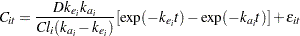where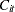is the observed concentration in groupat time,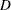is the dose of theophylline,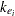is the elimination rate constant in group,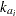is the absorption rate in group,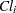is the clearance in group, and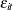denotes the model error. Because the rates and the clearance must be positive, you can parameterize the model in terms of log rates and the log clearance: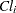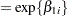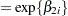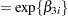In this parameterization the model contains six parameters, and the rates and clearance vary by group. The following PROC NLIN statements fit the model and obtain the group-specific parameter estimates:

proc nlin data=theop outest=cov;
parms beta1_1=-3.22 beta2_1=0.47 beta3_1=-2.45
beta1_2=-3.22 beta2_2=0.47 beta3_2=-2.45;
if (group=1) then do;
cl   = exp(beta1_1);
ka   = exp(beta2_1);
ke   = exp(beta3_1);
end; else do;
cl   = exp(beta1_2);
ka   = exp(beta2_2);
ke   = exp(beta3_2);
end;
mean = dose*ke*ka*(exp(-ke*time)-exp(-ka*time))/cl/(ka-ke);
model conc = mean;
ods output ParameterEstimates=ests;
run;

The conditional programming statements determine the clearance, elimination, and absorption rates depending on the value of the group variable. The OUTEST= option in the PROC NLIN statement saves estimates and their covariance matrix to the data set cov. The ODS OUTPUT statement saves the "Parameter Estimates" table to the data set ests.

Output 40.17.1 displays the analysis of variance table and the parameter estimates from this NLIN run. Note that the confidence levels in the "Parameter Estimates" table are based on 92 degrees of freedom, corresponding to the residual degrees of freedom in the analysis of variance table.

Output 40.17.1 Analysis of Variance and Parameter Estimates for Nonlinear Model
The NLIN Procedure

 Note: An intercept was not specified for this model.

Source DF Sum of Squares Mean Square F Value Approx
Pr > F
Model 6 3247.9 541.3 358.56 <.0001
Error 92 138.9 1.5097
Uncorrected Total 98 3386.8

Parameter Estimate Approx
Std Error
Approximate 95% Confidence
Limits
beta1_1 -3.5671 0.0864 -3.7387 -3.3956
beta2_1 0.4421 0.1349 0.1742 0.7101
beta3_1 -2.6230 0.1265 -2.8742 -2.3718
beta1_2 -3.0111 0.1061 -3.2219 -2.8003
beta2_2 0.3977 0.1987 0.00305 0.7924
beta3_2 -2.4442 0.1618 -2.7655 -2.1229

The following DATA step extracts the part of the cov data set that contains the covariance matrix of the parameter estimates in Output 40.17.1 and renames the variables as Col1–Col6. Output 40.17.2 shows the result of the DATA step.

data covb;
set cov(where=(_type_='COVB'));
rename beta1_1=col1 beta2_1=col2 beta3_1=col3
beta1_2=col4 beta2_2=col5 beta3_2=col6;
row = _n_;
Parm = 1;
keep parm row beta:;
run;
proc print data=covb;
run;

Output 40.17.2 Covariance Matrix of NLIN Parameter Estimates
Obs col1 col2 col3 col4 col5 col6 row Parm
1 0.007462 -0.005222 0.010234 0.000000 0.000000 0.000000 1 1
2 -0.005222 0.018197 -0.010590 0.000000 0.000000 0.000000 2 1
3 0.010234 -0.010590 0.015999 0.000000 0.000000 0.000000 3 1
4 0.000000 0.000000 0.000000 0.011261 -0.009096 0.015785 4 1
5 0.000000 0.000000 0.000000 -0.009096 0.039487 -0.019996 5 1
6 0.000000 0.000000 0.000000 0.015785 -0.019996 0.026172 6 1

The reason for this transformation of the data is to use the resulting data set to define a covariance structure in PROC GLIMMIX. The following statements reconstitute a model in which the parameter estimates from PROC NLIN are the observations and in which the covariance matrix of the "observations" matches the covariance matrix of the NLIN parameter estimates:

proc glimmix data=ests order=data;
class Parameter;
model Estimate = Parameter / noint df=92 s;
random _residual_ / type=lin(1) ldata=covb v;
parms (1) / noiter;
lsmeans parameter / cl;
lsmestimate Parameter
'beta1 eq. across groups' 1 0 0 -1,
'beta2 eq. across groups' 0 1 0  0 -1,
'beta3 eq. across groups' 0 0 1  0  0  -1 /
run;

In other words, you are using PROC GLIMMIX to set up a linear statistical model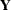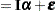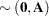where the covariance matrix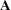is given by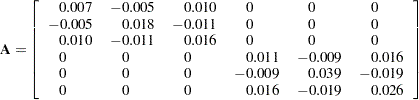The generalized least squares estimate for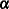in this saturated model reproduces the observations: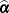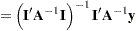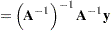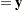The ORDER=DATA option in the PROC GLIMMIX statement requests that the sort order of the Parameter variable be identical to the order in which it appeared in the "Parameter Estimates" table of the NLIN procedure (Output 40.17.1). The MODEL statement uses the Estimate and Parameter variables from that table to form a model in which thematrix is the identity; hence the NOINT option. The DF=92 option sets the degrees of freedom equal to the value used in the NLIN procedure. The RANDOM statement specifies a linear covariance structure with a single component and supplies the values for the structure through the LDATA= data set. This structure models the covariance matrix as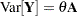, where thematrix is given previously. Essentially, the TYPE=LIN(1) structure forces an unstructured covariance matrix onto the data. To make this work, the parameter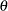is held fixed at 1 in the PARMS statement.

Output 40.17.3 displays the parameter estimates and least squares means for this model. Note that estimates and least squares means are identical, since thematrix is the identity. Also, the confidence limits agree with the values reported by PROC NLIN (see Output 40.17.1).

Output 40.17.3 Parameter Estimates and LS-Means from Summary Data
The GLIMMIX Procedure

Solutions for Fixed Effects
Effect Parameter Estimate Standard Error DF t Value Pr > |t|
Parameter beta1_1 -3.5671 0.08638 92 -41.29 <.0001
Parameter beta2_1 0.4421 0.1349 92 3.28 0.0015
Parameter beta3_1 -2.6230 0.1265 92 -20.74 <.0001
Parameter beta1_2 -3.0111 0.1061 92 -28.37 <.0001
Parameter beta2_2 0.3977 0.1987 92 2.00 0.0483
Parameter beta3_2 -2.4442 0.1618 92 -15.11 <.0001

Parameter Least Squares Means
Parameter Estimate Standard Error DF t Value Pr > |t| Alpha Lower Upper
beta1_1 -3.5671 0.08638 92 -41.29 <.0001 0.05 -3.7387 -3.3956
beta2_1 0.4421 0.1349 92 3.28 0.0015 0.05 0.1742 0.7101
beta3_1 -2.6230 0.1265 92 -20.74 <.0001 0.05 -2.8742 -2.3718
beta1_2 -3.0111 0.1061 92 -28.37 <.0001 0.05 -3.2219 -2.8003
beta2_2 0.3977 0.1987 92 2.00 0.0483 0.05 0.003050 0.7924
beta3_2 -2.4442 0.1618 92 -15.11 <.0001 0.05 -2.7655 -2.1229

The (marginal) covariance matrix of the data is shown in Output 40.17.4 to confirm that it matches thematrix given earlier.

Output 40.17.4 R-Side Covariance Matrix
Estimated V Matrix for Subject 1
Row Col1 Col2 Col3 Col4 Col5 Col6
1 0.007462 -0.00522 0.01023
2 -0.00522 0.01820 -0.01059
3 0.01023 -0.01059 0.01600
4       0.01126 -0.00910 0.01579
5       -0.00910 0.03949 -0.02000
6       0.01579 -0.02000 0.02617

The LSMESTIMATE statement specifies three linear functions. These set equal theparameters from the groups. The step-down Bonferroni adjustment requests a multiplicity adjustment for the family of three tests. The FTEST option requests a joint test of the three estimable functions; it is a global test of parameter homogeneity across groups.

Output 40.17.5 displays the result from the LSMESTIMATE statement. The joint test is highly significant (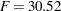,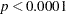). From the p-values associated with the individual rows of the estimates, you can see that the lack of homogeneity is due to group differences for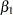, the log clearance.

Output 40.17.5 Test of Parameter Homogeneity across Groups
Least Squares Means Estimates
Effect Label Estimate Standard Error DF t Value Pr > |t| Adj P
Parameter beta1 eq. across groups -0.5560 0.1368 92 -4.06 0.0001 0.0003
Parameter beta2 eq. across groups 0.04443 0.2402 92 0.18 0.8537 0.8537
Parameter beta3 eq. across groups -0.1788 0.2054 92 -0.87 0.3862 0.7725

F Test for Least Squares Means Estimates
Label Num DF Den DF F Value Pr > F
Homogeneity 3 92 30.52 <.0001

An alternative method to set up this model is given by the following statements, where the data set pdata contains the covariance parameters:

random _residual_ / type=un;
parms / pdata=pdata noiter

The following DATA step creates an appropriate PDATA= data set from the data set covb constructed earlier:

data pdata; set covb;
array col{6};
do i=1 to _n_;
estimate = col{i};
output;
end;
keep estimate;
run;